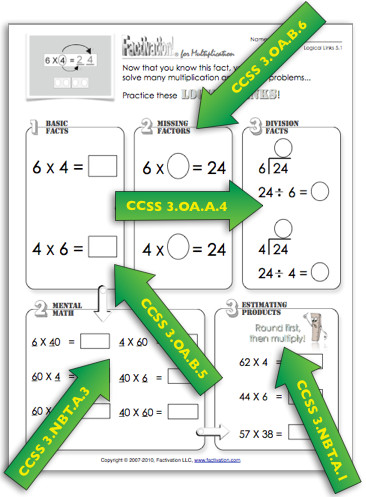##### Print or Share Flyer## 21 Aug Factivation!® Aligns to State Standards

### Aligned to CCSS and Other State Standards!

Factivation!® ensures fluency while also providing educators with a variety of accompanying resources that promote in-depth examination of facts. All CCSS 1st-3rd grade Operations and Algebraic Thinking standards are addressed multiple times throughout each lesson, as well as some 4th grade skills. The arrows below indicate the Common Core standards presented within one “Logical Links” activity page. There are a variety of printable options with each lesson! The printables may be copied or projected for class discussion. (iPad classrooms may download and complete them using a PDF app, if preferred.) We invite you to sample some of the other CCSS-aligned printables in any of our FREE lessons (see lesson menus, left).[pinit]#### Sample Printable from the Factivation!® Program

This Logical Links printable (left) is from Lesson 5 of the Factivation!® for Multiplication program. Teachers utilizing this power printable provide students with repeated reinforcement in the following 3rd grade CCSS Operations and Algebraic Thinking skills:
3.OA.A.4
Represent and solve problems involving multiplication and division-
Determine the unknown whole number in a multiplication or division equation relating three whole numbers.
3.OA.B.5
Understand properties of multiplication and the relationship between multiplication and division-
Apply properties of operations as strategies to multiply and divide. Examples: If 6 × 4 = 24 is known, then 4 × 6 = 24 is also known. (Commutative property of multiplication)…

3.OA.B.6
Understand properties of multiplication and the relationship between multiplication and division-
Understand division as an unknown-factor problem.

The following Number & Operations in Base Ten standards are also addressed:
3.NBT.A.1
Use place value understanding and properties of operations to perform multi-digit arithmetic-
Use place value understanding to round whole numbers to the nearest 10 or 100.
3.NBT.A.3
Use place value understanding and properties of operations to perform multi-digit arithmetic-
Multiply one-digit whole numbers by multiples of 10 in the range 10–90 (e.g., 9 × 80, 5 × 60) using strategies based on place value and properties of operations.

#### Rigorous Examination of Individual Facts and the Relationship of Multiplication to Division

The Logical Links printables are just one of many CCSS-aligned activity pages accompanying each Factivation!® lesson! There are three Logical Links pages with each of the nine Multiplication/Division lessons, so students will be exposed to the above five CCSS standards 27 times throughout the course of the Factivation!® program, each time working intensely with a different Multiplication/Division fact family. (Logical Links printables are also available with the Addition/Subtraction program.)

TEKS (Texas)

OAS (Oklahoma)
Coming Soon

SUBMIT REQUEST